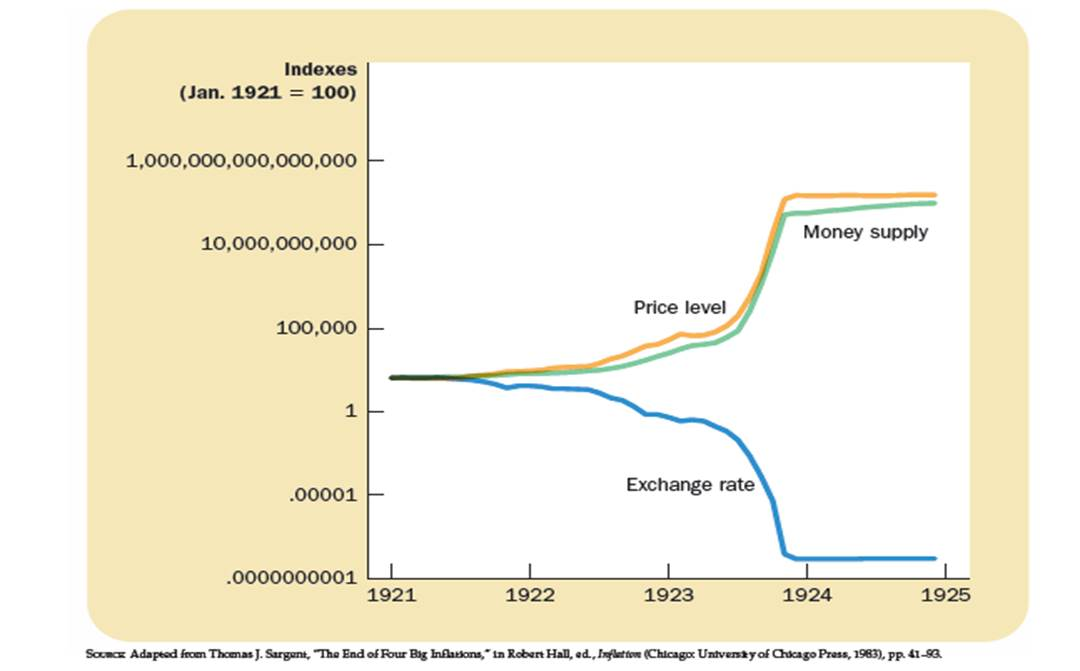### 12.3 The Harrod-Balassa-Samuelson model

It is an empirically well-established fact that countries that are in the process of becoming industrialized countries have higher inflation rates than industrialized countries. This fact was much debated when it came to the criteria for the accession to the Euro, especially for the CEE countries. The argument of one side was that (too) high inflation rates were due to an overly expansive monetary policy. The argument of the other side was that higher inflation rates in emerging markets were mainly due to their higher growth rates. The Harrod-Balassa-Samuelson model explains this correlation.

The model considers two countries, A and B, whose economies consist of two sectors, tradable (T) and non-tradable (NT) goods. For tradable goods, the "law of one price" applies, i.e. ${p}_{T,B}>{p}_{T,A}$. Let country A be the emerging market, country B the industrialized country, i.e. productivity is the same in both countries in the NT sector ($\mathit{GP}{A}_{\mathit{NT},A}=\mathit{GP}{A}_{\mathit{NT},B}=1$, GPA is the marginal product of labor), in the T sector country B is more productive ($\mathit{GP}{A}_{T,A}<\mathit{GP}{A}_{T,B}$). In both countries, the labor markets are complete, i.e. all workers are paid according to the marginal revenue product. Wages are equal between sectors, and workers can move between sectors. Therefore,

${p}_{T}\cdot \mathit{GP}{A}_{T,A}={w}_{A}={p}_{\mathit{NT},A}\cdot \mathit{GP}{A}_{\mathit{NT},A}={p}_{\mathit{NT},A},$

where $p$ describes the price of tradable or non-tradable goods, and $w$ the wage rate of the respective country. The same equation applies to country B. Since the productivity in the tradable sector in country B is higher than in country A, the wages ${w}_{B}>{w}_{A}$ are, of course, also higher. But since productivity in the non-tradable sector is the same in A and B, the price in the non-tradable sector and therefore the total price level in B must also be higher (${p}_{\mathit{NT},B}>{p}_{\mathit{NT},A}$ and therefore ${p}_{B}>{p}_{A}$). Thus, country B is richer, has higher wages and a higher price level (Penn effect).

Now we assume that country A has higher productivity growth in tradable goods, i.e. the emerging market is catching up with the industrialized countries. To simplify matters, country B is in steady state equilibrium. If productivity in the tradable sector rises in country A, wages in the tradable sector will also rise as a result of remuneration according to the marginal revenue product. Because the labor markets are complete, wages in the non-tradable sector also rise. However, since productivity in the non-tradable sector is constant, the price of the non-tradable goods must increase and with it the total price level. Thus, the inflation rate is higher in the emerging market than in the industrialized country due to the productivity growth.

Empirical evidence on purchasing power parity theory

The purchasing power parity theory explains exchange rate movements relatively well for country pairs with very high inflation differences, but cannot be verified for countries with low inflation. For example, for periods of hyperinflation, like in Germany between the world wars, the parallel development of price level and exchange rate can be observed very well.The law of uniform prices does not apply empirically if the deviations are too small, because transport costs, for example, limit the arbitrage possibilities. Nevertheless, there is a weak relationship between exchange rate changes and inflation differences in both the short and medium term, i.e. there is a positive correlation between real and nominal exchange rate. Due to price inertia, the high short-term volatility of the nominal exchange rate is transmitted to the real exchange rate.(c) by Christian Bauer
Prof. Dr. Christian Bauer
Chair of monetary economics
Trier University
D-54296 Trier
Tel.: +49 (0)651/201-2743
E-mail: Bauer@uni-trier.de
URL: https://www.cbauer.de